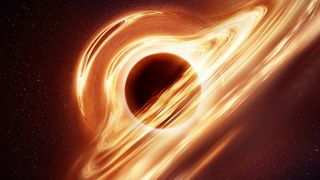# What is a singularity?To understand what a singularity is, imagine the force of gravity compressing you down into an infinitely tiny point, so that you occupy literally no volume. That sounds impossible … and it is. These "singularities" are found in the centers of black holes and at the beginning of the Big Bang. These singularities don't represent something physical. Rather, when they appear in mathematics, they are telling us that our theories of physics are breaking down, and we need to replace them with a better understanding.

## What is a singularity?

Singularities can happen anywhere, and they are surprisingly common in the mathematics that physicists use to understand the universe. Put simply, singularities are places where the mathematics "misbehave," typically by generating infinitely large values. There are examples of mathematical singularities throughout physics: Typically, any time an equation uses 1/X, as X goes to zero, the value of the equation goes to infinity.

Most of these singularities, however, can usually be resolved by pointing out that the equations are missing some factor, or noting the physical impossibility of ever reaching the singularity point. In other words, they are probably not "real."

But there are singularities in physics that do not have simple resolutions. The most famous are gravitational singularities, the infinities that appear in Einstein's general relativity (GR), which is currently our best theory of how gravity works.

In general relativity, there are two kinds of singularities: coordinate singularities and true singularities. Coordinate singularities happen when an infinity appears in one coordinate system (a particular choice for recording separations in time and space) but disappears in another.

For example, the physicist Karl Schwarzschild applied general relativity to the simple system of a spherical mass, such as a star. He found that the solution contained two singularities, one in the very center and one at a certain distance from the center, known today as the Schwarzschild radius. For many years, physicists thought that both singularities signaled breakdowns in the theory, but it didn't matter as long as the radius of the spherical mass was larger than the Schwarzschild radius. All physicists needed was for GR to predict the gravitational influence outside the mass, according to San Jose State University.

But what would happen if an object were squeezed below its own Schwarzschild radius? Then that singularity would be outside the mass, and it would mean that GR is breaking down in a region that it shouldn't.

It was soon discovered that the singularity at the Schwarzschild radius was a coordinate singularity. A change in coordinate systems removes the singularity, saving GR and allowing it to still make valid predictions, astrophysicist Ethan Siegel writes in Forbes.

## Where do gravitational singularities happen?

But the singularity at the centers of spherical masses remained. If you squeeze an object below its Schwarzschild radius, then its own gravity becomes so intense that it just keeps on squeezing all by itself, all the way down to an infinitely tiny point, according to National Geographic.

For decades physicists debated whether a collapse to an infinitely tiny point was possible, or whether some other force was able to prevent total collapse. While white dwarfs and neutron stars can hold themselves up indefinitely, any object larger than about six times the mass of the sun will have too much gravity, overwhelming all the other forces and collapsing into an infinitely tiny point: a true singularity, according to NASA.

## What are naked singularities?

These are what we call the black holes: a point of infinite density, surrounded by an event horizon located at the Schwarzschild radius. The event horizon "protects" the singularity, preventing outside observers from seeing it unless they traverse the event horizon, according to Quanta Magazine.

Physicists long thought that in GR, all singularities like this are surrounded by event horizons, and this concept was known as the Cosmic Censorship Hypothesis — so named because it was surmised that some process in the universe prevented (or "censored") singularities from being viewable. However, computer simulations and theoretical work have raised the possibility of exposed (or "naked") singularities. A naked singularity would be just that: a singularity without an event horizon, fully observable from the outside universe. Whether such exposed singularities exist continues to be a subject of considerable debate.

## What's really at the center of a black hole?

Because they are mathematical singularities, nobody knows what's really at the center of a black hole. To understand it, we need a theory of gravity beyond GR. Specifically, we need a quantum theory of gravity, one that can describe the behavior of strong gravity at very tiny scales, according to Physics of the Universe.

Hypotheses that modify or replace general relativity to give us a replacement of the black hole singularity include Planck stars (a highly-compressed exotic form of matter), gravastars (a thin shell of matter supported by exotic gravity), and dark energy stars (an exotic state of vacuum energy that behaves like a black hole). To date, all these ideas are hypothetical, and a true answer must await a quantum theory of gravity.